CBSE Class 10 Sample Paper for 2021 Boards - Maths Standard

Class 10
Solutions of Sample Papers for Class 10 Boards

## In the figure, ABCD is a square of side 14 cm. Semi-circles are drawn with each side of square as diameter. Find the area of the shaded region.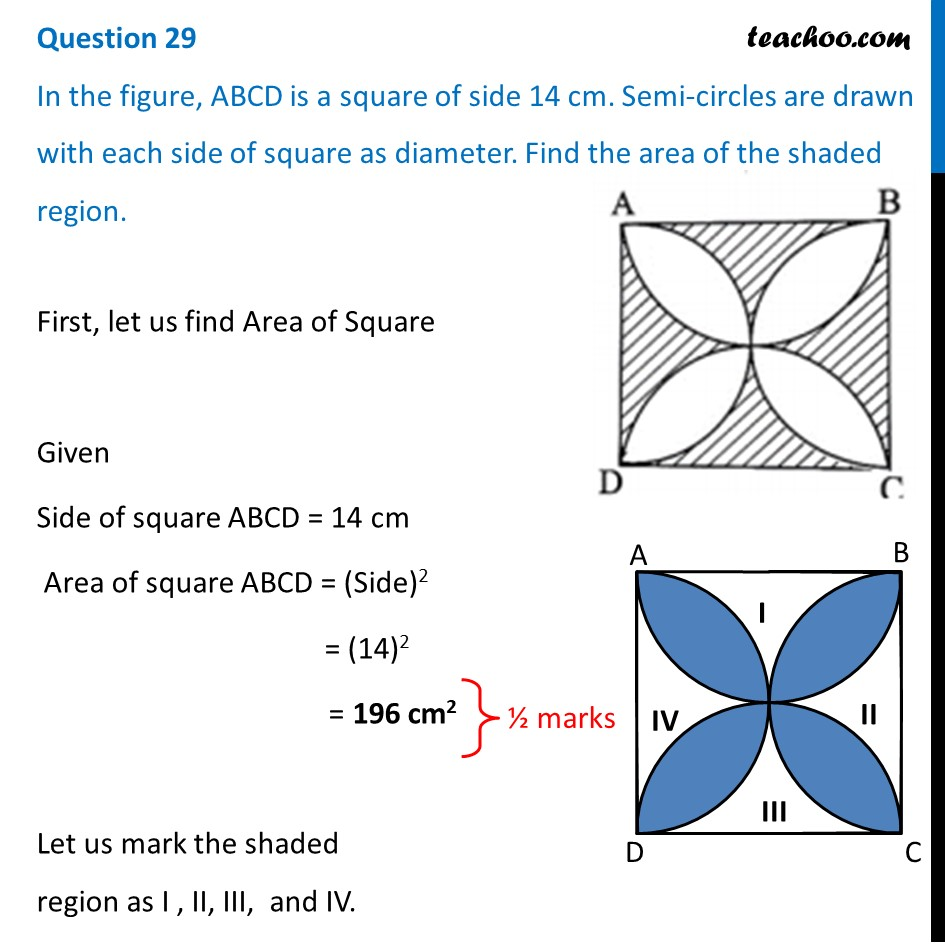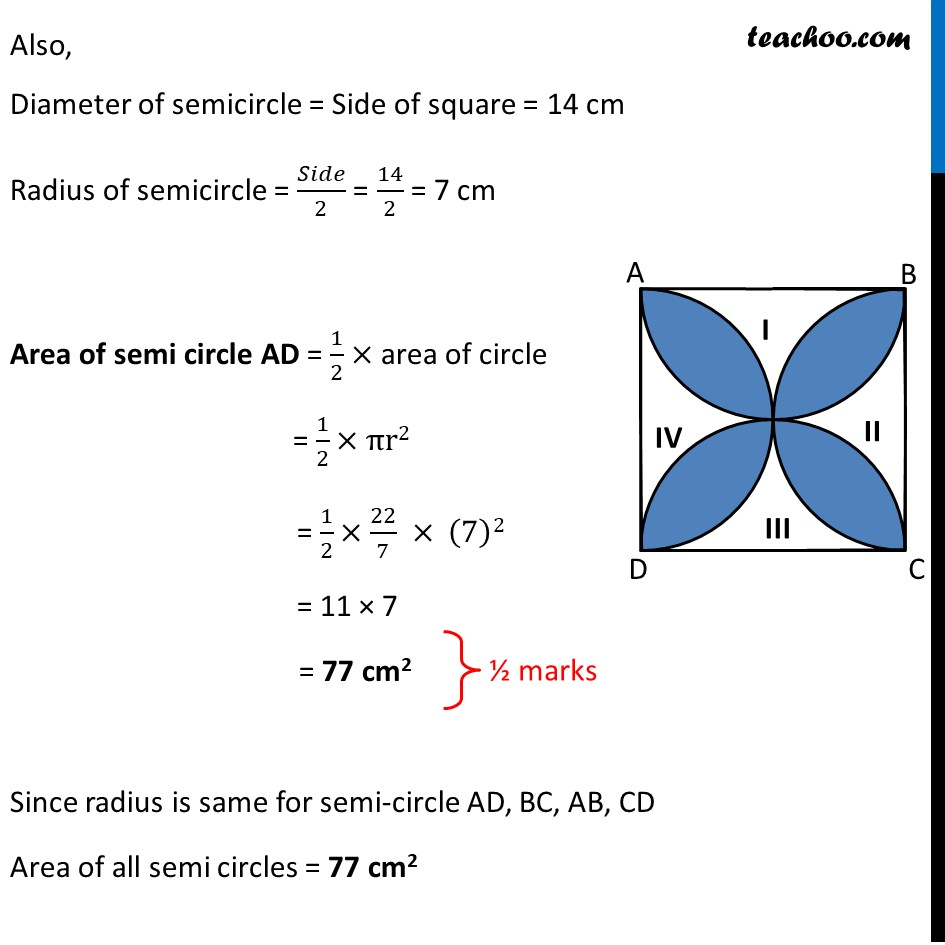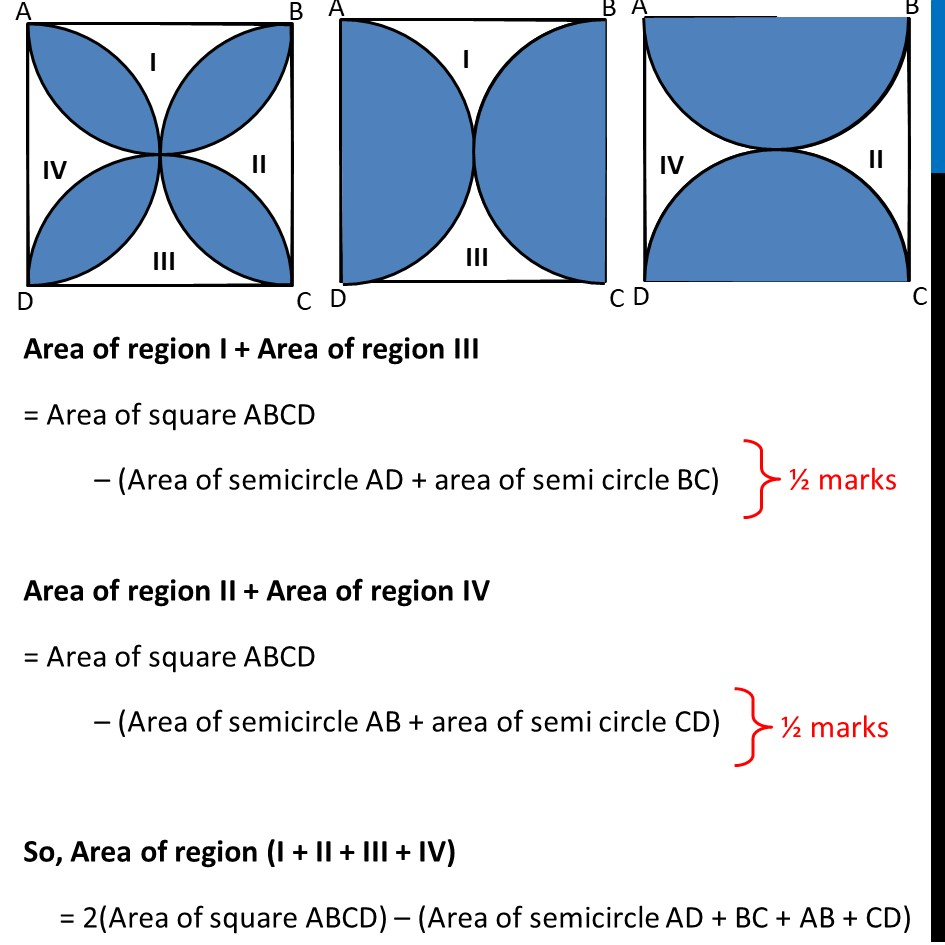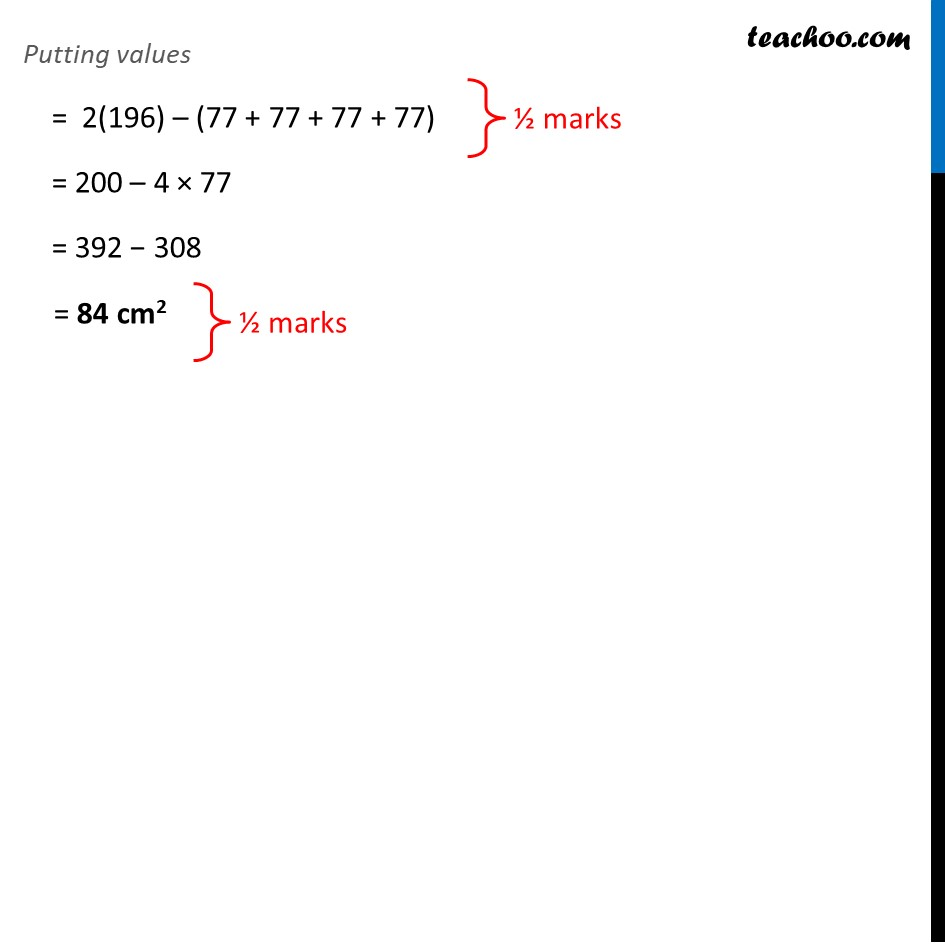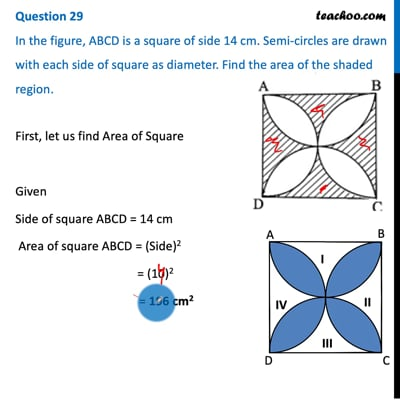This video is only available for Teachoo black users

Note : This is similar to Example 6 of NCERT – Chapter 12 Class 10

Introducing your new favourite teacher - Teachoo Black, at only ₹83 per month

### Transcript

Question 29 In the figure, ABCD is a square of side 14 cm. Semi-circles are drawn with each side of square as diameter. Find the area of the shaded region. First, let us find Area of Square Given Side of square ABCD = 14 cm Area of square ABCD = (Side)2 = (14)2 = 196 cm2 Let us mark the shaded region as I , II, III, and IV. Also, Diameter of semicircle = Side of square = 14 cm Radius of semicircle = 𝑆𝑖𝑑𝑒/2 = 14/2 = 7 cm Area of semi circle AD = 1/2× area of circle = 1/2×πr2 = 1/2×22/7 × (7)2 = 11 × 7 = 77 cm2 Since radius is same for semi-circle AD, BC, AB, CD Area of all semi circles = 77 cm2 Area of region I + Area of region III = Area of square ABCD – (Area of semicircle AD + area of semi circle BC) Area of region II + Area of region IV = Area of square ABCD – (Area of semicircle AB + area of semi circle CD) So, Area of region (I + II + III + IV) = 2(Area of square ABCD) – (Area of semicircle AD + BC + AB + CD) Putting values = 2(196) – (77 + 77 + 77 + 77) = 200 – 4 × 77 = 392 − 308 = 84 cm2